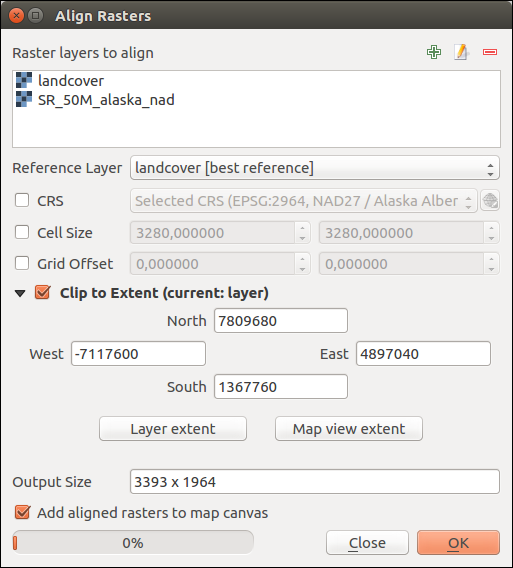# 15.2. Raster Analysis¶

## 15.2.1. Raster Calculator¶

The Raster Calculator in the Raster menu allows you to perform calculations on the basis of existing raster pixel values (see figure_raster_calculator). The results are written to a new raster layer with a GDAL-supported format.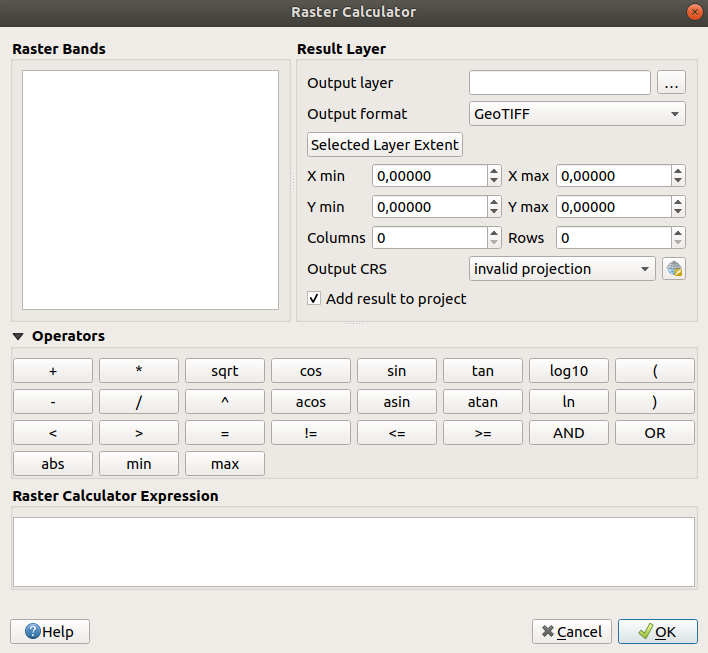The Raster bands list contains all loaded raster layers that can be used. To add a raster to the raster calculator expression field, double click its name in the Fields list. You can then use the operators to construct calculation expressions, or you can just type them into the box.

In the Result layer section, you will need to define an output layer. You can then define the extent of the calculation area based on an input raster layer, or based on X,Y coordinates and on columns and rows, to set the resolution of the output layer. If the input layer has a different resolution, the values will be resampled with the nearest neighbor algorithm.

The Operators section contains all available operators. To add an operator to the raster calculator expression box, click the appropriate button. Mathematical calculations (`+`, `-`, `*`, ... ) and trigonometric functions (`sin`, `cos`, `tan`, ... ) are available. Conditional expressions (`=`, `!=`, `<`, `>=`, ... ) return either 0 for false or 1 for true, and therefore can be used with other operators and functions. Stay tuned for more operators to come!

With theAdd result to project checkbox, the result layer will automatically be added to the legend area and can be visualized.

### 15.2.1.1. Examples¶

Convert elevation values from meters to feet

Creating an elevation raster in feet from a raster in meters, you need to use the conversion factor for meters to feet: 3.28. The expression is:

```"[email protected]" * 3.28
```

If you want to mask out parts of a raster -- say, for instance, because you are only interested in elevations above 0 meters -- you can use the following expression to create a mask and apply the result to a raster in one step.

```("[email protected]" >= 0) * "[email protected]"
```

In other words, for every cell greater than or equal to 0 the conditional expression evaluates to 1, which keeps the original value by multiplying it by 1. Otherwise the conditional expression evaluates to 0, which sets the raster value to 0. This creates the mask on the fly.

If you want to classify a raster -- say, for instance into two elevation classes, you can use the following expression to create a raster with two values 1 and 2 in one step.

```("[email protected]" < 50) * 1 + ("[email protected]" >= 50) * 2
```

In other words, for every cell less than 50 set its value to 1. For every cell greater than or equal 50 set its value to 2.

## 15.2.2. Raster Alignment¶

This tool is able to take several rasters as input and to align them perfectly, that means:

• reproject to the same CRS,

• resample to the same cell size and offset in the grid,

• clip to a region of interest,

• rescale values when required.

All rasters will be saved in another files.

First, open the tools from Raster ▶ Align Raster... and click on the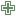Add new raster button to choose one existing raster in QGIS. Select an output file to save the raster after the alignment, the resampling method and if the tools need to Rescale values according to the cell size. The resampling method can be (see figure_raster_align_edit):

• Nearest Neighbor

• Bilinear (2x2 kernel)

• Cubic (4x4 kernel): Cubic Convolution Approximation

• Cubic B-Spline (4x4 kernel): Cubic B-Spline Approximation

• Lanczos (6x6 kernel): Lanczos windowed sinc interpolation

• Average: computes the average of all non-NODATA contributing pixels

• Mode: selects the value which appears most often of all the sampled points

• Maximum, Minimum, Mediane, First Quartile (Q1) or Third Quartile (Q3) of all non-NODATA contributing pixels

Methods like maximum, minimum, mediane, first and third quartiles are available only if QGIS is built with GDAL >= 2.0.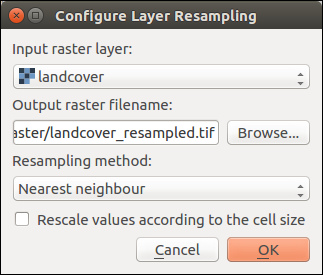In the main Align raster dialog, you can still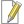Edit file settings or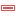Remove an existing file from the list of raster layers. You can also choose one or more other options (see figure_raster_align):

• Select the Reference Layer,

• Transform into a new CRS,

• Setup a different Cell size,

• Setup a different Grid Offset,

• Clip to Extent: it can be user-defined or based on a layer or the map view

• Output Size,

• Add aligned raster to the map canvas.